# Fourier transform of Python OpenCV image processing, lesson 52

2022-01-30 13:20:11

Little knowledge , Great challenge ！ This article is participating in 「 A programmer must have a little knowledge 」 Creative activities

This article has participated in  「 Digging force Star Program 」 , Win a creative gift bag , Challenge creation incentive fund .

Python OpenCV 365 Day study plan , Go into the field of image with the eraser . This blog is the third in this series 52 piece .

The Fourier transform （Fourier Transform,FT） For the first time today , Follow the old routine , We still apply it first , Then step by step iterative learning .

Fourier transform is right The same thing The change of viewing angle , No longer viewing from the time domain , View from the frequency domain . There are two new words mentioned here , Time domain and frequency domain , Let's briefly understand , Time domain , Changes in things over time , Frequency domain refers to the change frequency of things , Is it very winding , No problem , First use , First tune API.

Fourier principle omits , Let's talk about the impact on the image after applying it .

• After using the high pass filter , Will retain high-frequency information , Enhance image detail , For example, boundary enhancement ;
• After using low-pass filter , Low frequency information will be retained , The border is blurred .

## Fourier transform application

Now that the principle has been put down first , Then apply it first , We will study separately numpy and OpenCV Fourier transform is realized in two ways .

### Numpy Fourier transform

adopt numpy The function used to realize the Fourier transform is `np.fft.fft2` , The function prototype is as follows ：

``````fft2(a, s=None, axes=(-2, -1), norm=None)
Copy code ``````

The parameters are described as follows ：

• `a`： The input image ;
• `s`： Sequence of integers , Size of output array ;
• `axex`： Sequence of integers , Used to calculate `FFT` The optional shaft of ;
• `norm`： Normalization model .

Some parameters, if not actually used , It's hard to see the results , Of course, the description of the function , still The official manual Most reliable . The coding process at the application level is as follows ： adopt `np.fft.fft2` The frequency distribution is obtained by Fourier transform of function , Call again `np.fft.fftshift` Function to transfer the center to the middle .

The test code is as follows ：

``````import cv2 as cv
import numpy as np
from matplotlib import pyplot as plt

img = cv.imread('test.jpg', 0)

#  Fast Fourier transform algorithm to get the frequency distribution , Transform the spatial domain into the frequency domain
f = np.fft.fft2(img)

#  The default result center point position is in the upper left corner , Transfer the center point to the middle position by the following code
#  Move the low frequency part to the center of the image
fshift = np.fft.fftshift(f)

# fft  The result is a complex number ,  The absolute result is the amplitude
result = 20*np.log(np.abs(fshift))

plt.subplot(121)
plt.imshow(img, cmap='gray')
plt.title('original')
plt.axis('off')

plt.subplot(122)
plt.imshow(result, cmap='gray')
plt.title('result')
plt.axis('off')

plt.show()
Copy code ``````

This is a case repeatedly mentioned in many places , In addition to the relevant contents . `np.fft.fft2` Is a frequency conversion function , Its first parameter is the input image , Gray scale image . The second parameter is optional , It determines the size of the output array . If the size of the second parameter is larger than the size of the input image , It's calculating FFT Before zero fill input image ; If it's smaller than the input image , Input the cut into the image . If no parameters are passed , The size of the output array will be the same as the size of the input , Test the following ：

``````import cv2 as cv
import numpy as np
from matplotlib import pyplot as plt

#  Create a picture
src = np.ones((5, 5), dtype=np.uint8)*100

print(src)
print(src.shape)

f = np.fft.fft2(src,(7,7))
print(f.shape)
print(f)
Copy code ``````

After the center point moves , You can refer to the running results in the figure below , And we can know `np.fft.fft2` After the function is applied, the complex matrix is obtained .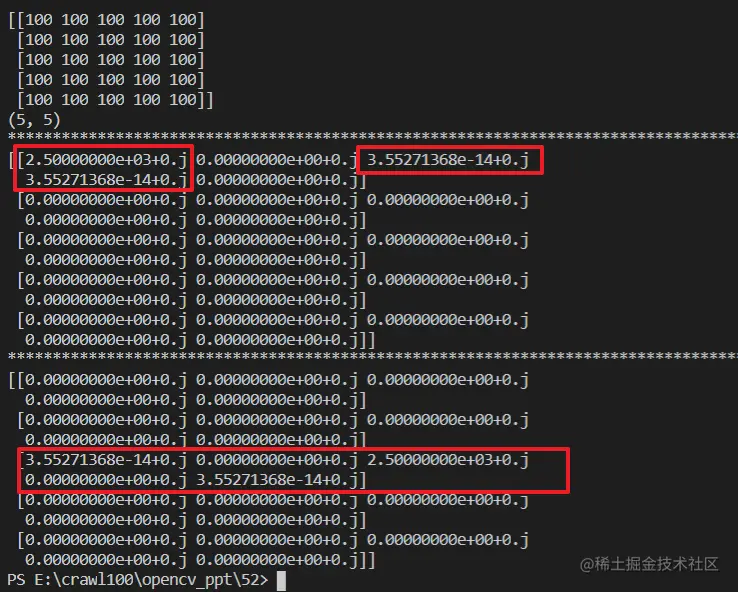Operations to be carried out later , Have some knowledge of Mathematics , I don't understand yet , Below are ： After frequency conversion , You can build the amplitude spectrum , Finally, the range is compressed by logarithmic transformation . Or it can be understood that the complex number is converted into floating-point number for Fourier spectrum display , The supplementary code is as follows ：

``````fimg = np.log(np.abs(fshift))
Copy code ``````

The final result is as follows , Of course, this is a random graph , If you change to a gray image , It can be verified as follows .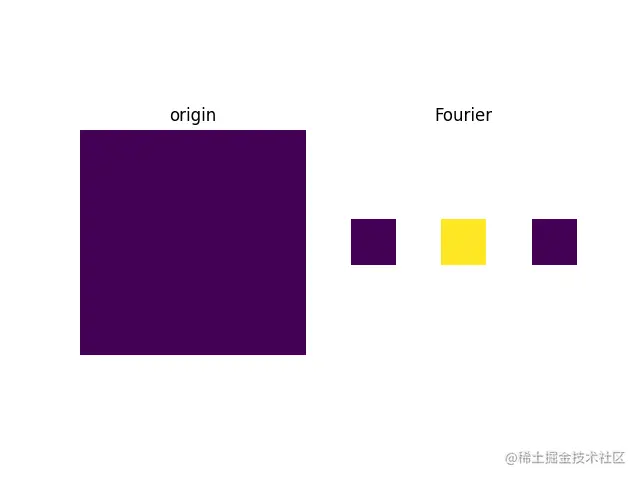The modified code is as follows ：

``````import cv2 as cv
import numpy as np
from matplotlib import pyplot as plt

#  Create a picture
# src = np.ones((5, 5), dtype=np.uint8)*100
src = cv.imread("./test.jpg", 0)
print("*"*100)
print(src)
print(src.shape)

f = np.fft.fft2(src)
print("*"*100)
print(f)

fshift = np.fft.fftshift(f)
print("*"*100)
print(fshift)
#  Convert complex numbers into floating-point numbers for Fourier spectrum display
fimg = 20*np.log(np.abs(fshift))
print(fimg)

#  Image display
plt.subplot(121), plt.imshow(src, "gray"), plt.title('origin')
plt.axis('off')
plt.subplot(122), plt.imshow(fimg, "gray"), plt.title('Fourier')
plt.axis('off')
plt.show()
Copy code ``````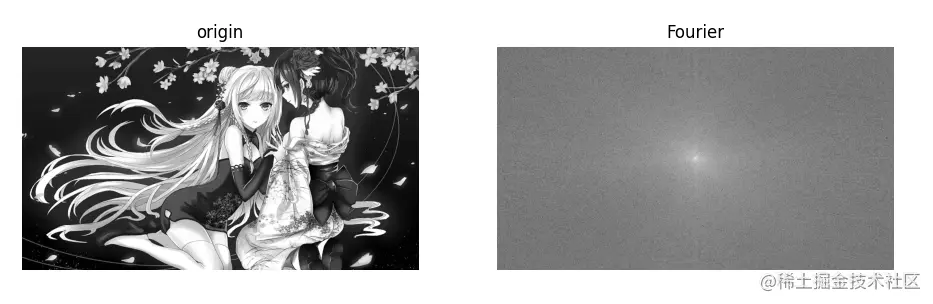Basic conclusion ： On the left is the original grayscale image , On the right is the frequency distribution map , The closer it is to the center The lower the frequency , The higher the gray value, the brighter the brightness, and the greater the amplitude of the signal representing this frequency . Next, inverse transform it , That is, reverse operation back , adopt `numpy` Realize inverse Fourier transform , It's the inverse of Fourier transform , take Spectrum image Convert to original image . The core functions and prototypes used are as follows . np.fft.ifft2

``````#  Realize the inverse Fourier transform of image , Returns a complex array
np.fft.ifft2(a, s=None, axes=(-2, -1), norm=None)
Copy code ``````

np.fft.ifftshift

``````# fftshit() The inverse of a function , It moves the center low frequency part of the spectrum image to the upper left corner
np.fft.ifftshift(x, axes=None)
Copy code ``````

According to the above , Reverse the Fourier transform , The test code is as follows ：

``````import cv2 as cv
import numpy as np
from matplotlib import pyplot as plt

#  Create a picture
# src = np.ones((5, 5), dtype=np.uint8)*100
src = cv.imread("./test.jpg", 0)
print("*"*100)
print(src)
print(src.shape)

f = np.fft.fft2(src)
print("*"*100)
print(f)

fshift = np.fft.fftshift(f)
print("*"*100)
print(fshift)

#  Convert complex numbers into floating-point numbers for Fourier spectrum display
fimg = np.log(np.abs(fshift))
print(fimg)

#  Inverse Fourier transform
ifshift = np.fft.ifftshift(fshift)
#  Convert complex numbers into floating-point numbers for Fourier spectrum display
ifimg = np.log(np.abs(ifshift))
if_img = np.fft.ifft2(ifshift)

origin_img = np.abs(if_img)

#  Image display
plt.subplot(221), plt.imshow(src, "gray"), plt.title('origin')
plt.axis('off')
plt.subplot(222), plt.imshow(fimg, "gray"), plt.title('fourier_img')
plt.axis('off')
plt.subplot(223), plt.imshow(origin_img, "gray"), plt.title('origin_img')
plt.axis('off')
plt.subplot(224), plt.imshow(ifimg, "gray"), plt.title('ifimg')
plt.axis('off')
plt.show()
Copy code ``````

The final result is as follows ：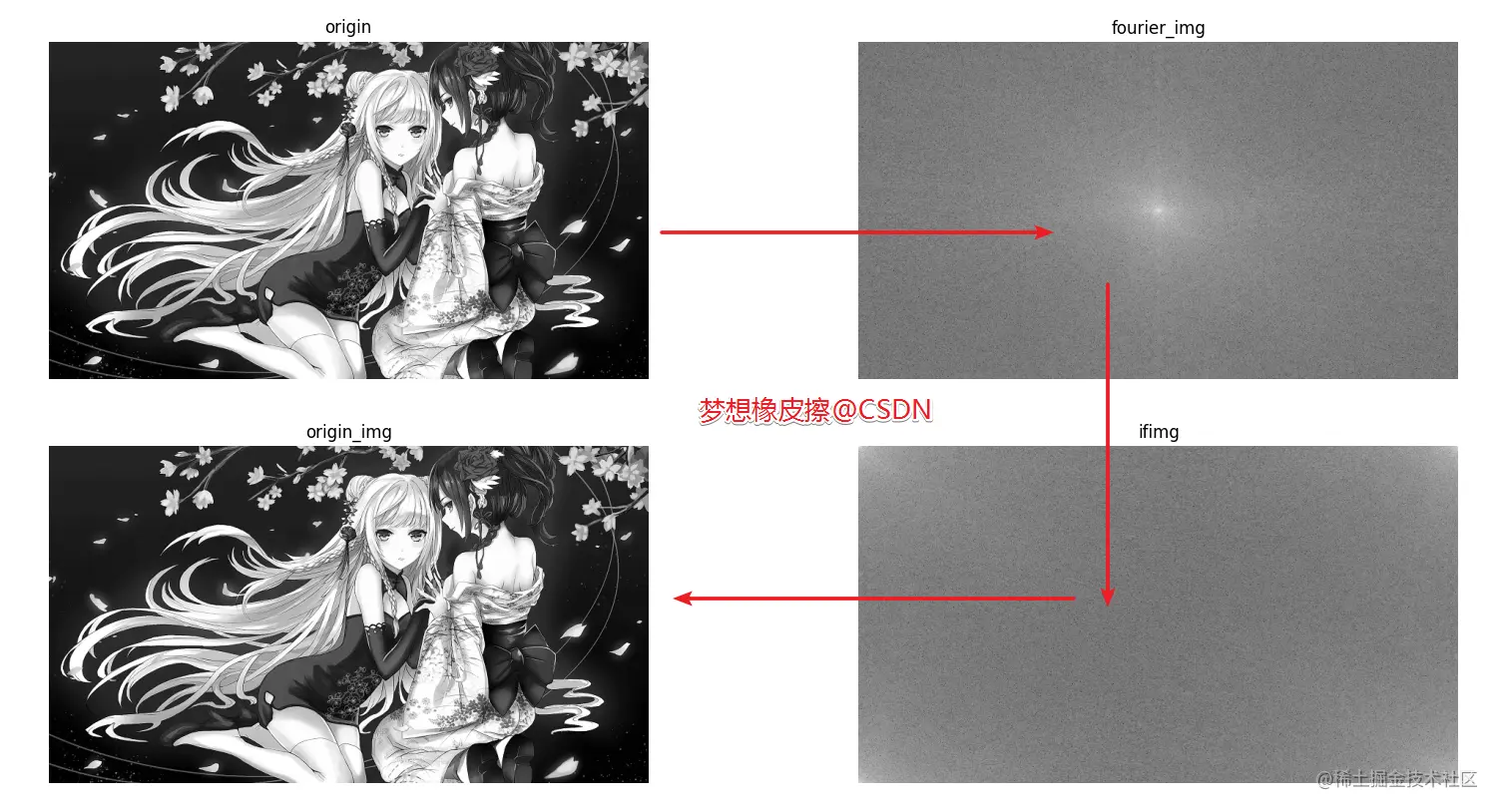If in the spectrum image after the above Fourier transform , Add a low-pass filter , We will get the following results .

``````import cv2 as cv
import numpy as np
from matplotlib import pyplot as plt

#  Realize low-pass filtering of Fourier transform
src = cv.imread("./test.jpg", 0)
f = np.fft.fft2(src)
fshift = np.fft.fftshift(f)

rows, cols = src.shape
crow, ccol = rows//2, cols//2

#  Make a mask , It's the same size as the image ,np.zeros initialization
mask = np.zeros((rows, cols), np.uint8)
#  Make the center position , Up and down, left and right 30, Set as 1
mask[crow-30:crow+30, ccol-30:ccol+30] = 1

#  Mask and  DFT  Only the middle region is preserved in the product multiplication

#  Inverse Fourier transform
ifshift = np.fft.ifftshift(fshift)
#  Convert complex numbers into floating-point numbers for Fourier spectrum display
ifimg = np.fft.ifft2(ifshift)
dft_img = np.abs(ifimg)

#  Image display
plt.subplot(121), plt.imshow(src, "gray"), plt.title('origin')
plt.axis('off')
plt.subplot(122), plt.imshow(dft_img, "gray"), plt.title('dft_img')
plt.axis('off')
plt.show()
Copy code ``````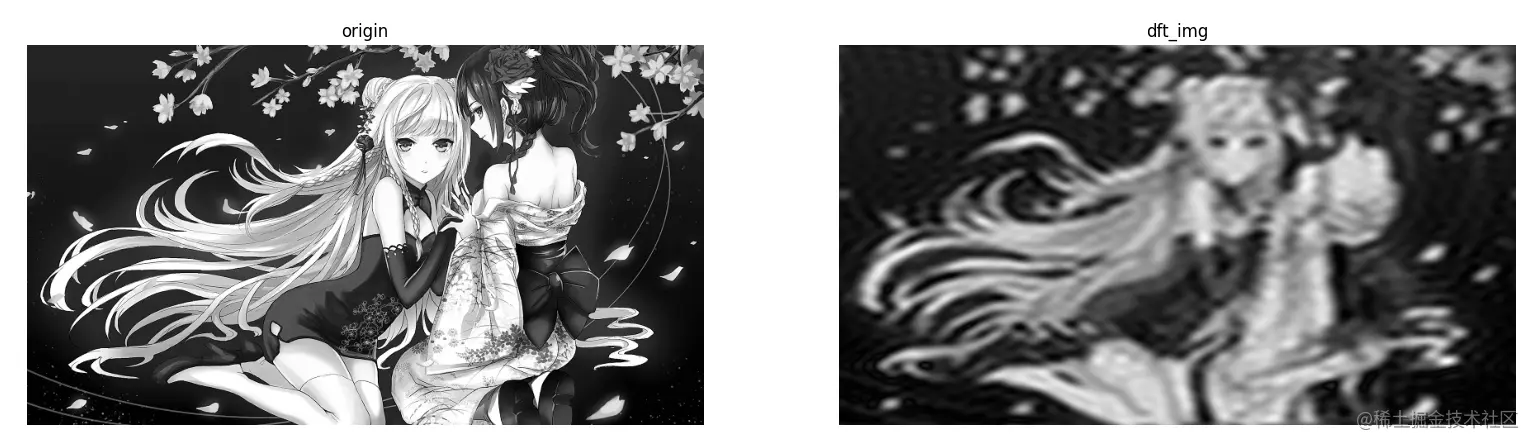If you want to achieve high pass filtering , Just modify the mask data .

``````mask = np.ones((rows, cols), np.uint8)
mask[crow-30:crow+30, ccol-30:ccol+30] = 0
Copy code ``````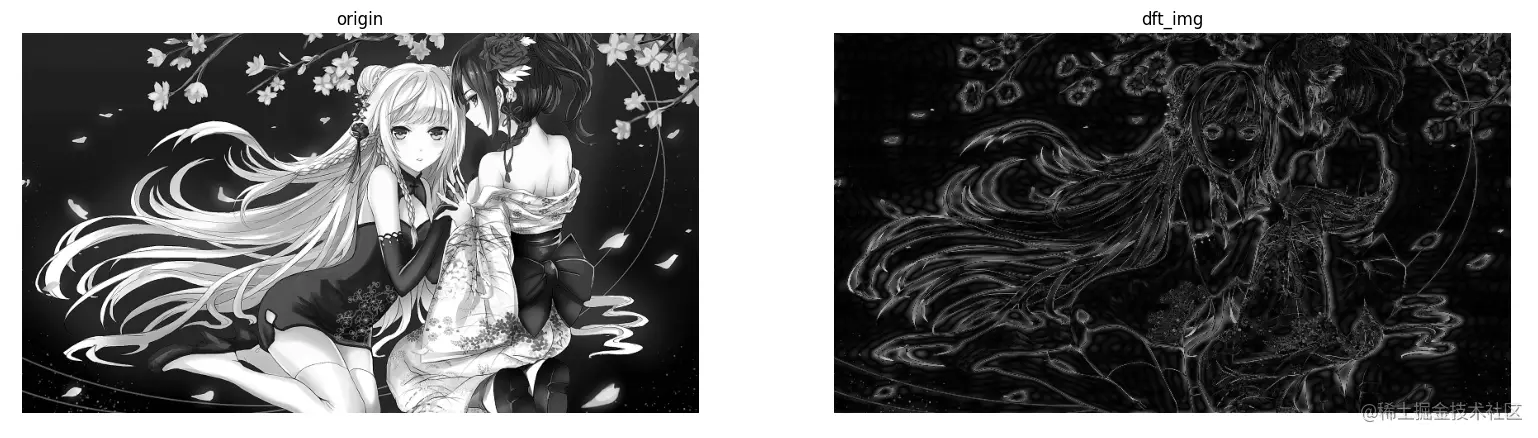I've read a lot of articles , But an article is mentioned repeatedly , Here is also a note ：

zhuanlan.zhihu.com/p/19763358 Of course, the content of Fourier transform in the official manual also needs a long time to read , Official website address

### OpenCV Fourier transform

OpenCV Realize Fourier transform and numpy Almost the same , The core difference is the use of functions , The differences are as follows ： Opencv Mainly through `cv2.dif` and `cv2.idif`（ Inverse Fourier transform ）, Before inputting an image, you need to convert the image from `np.uint8` Convert to `np.float32` Format , The frequency of the result is 0 It's going to be in the upper left corner , To switch to the center position , adopt `shift` Transform to achieve , And numpy Agreement ,`cv2.dif` The result returned is dual channel （ Real component , Imaginary part ）, It also needs to be converted into image format to show .

function cv2.dft() The prototype is as follows

``````dst = cv.dft(src[, dst[, flags[, nonzeroRows]]])
Copy code ``````

Parameter description ：

• `src`： The input image , requirement `np.float32` Format ;
• `dst`： Output image , Two channels （ Real component + Imaginary part ）, The size and type depend on the third parameter `flags`;
• `flags`： Represents a conversion token , The default is 0, There are multiple values , See later ;
• `nonzeroRows`： The default is 0, Not for now .

`flags` The values are as follows ：

• `DFT_INVERSE`： Replace the default forward transform with one-dimensional or two-dimensional inverse transform ;
• `DFT_SCALE`： Scale identifier , Calculate the scaling result according to the average number of data elements , if there be N Elements , Then the output result is in 1/N Zoom output , Often with `DFT_INVERSE` Use it with ;
• `DFT_ROWS`： Forward or reverse Fourier transform is performed on each row of the input matrix ; This identifier can be used to reduce the cost of resources when dealing with a variety of appropriate , These processes are often complex operations such as three-dimensional or high-dimensional transformation ;
• `DFT_COMPLEX_OUTPUT`： Forward transform one-dimensional or two-dimensional real arrays , Although this result is a complex array , But it has conjugate symmetry of the complex number （CCS）, It can be filled with an array of real numbers with the same size as the original array , This is the fastest choice and the default method of the function . You may want to get a full-size complex array （ Like simple spectral analysis and so on ）, By setting the flag bit, the function can generate a full-size complex output array ;
• `DFT_REAL_OUTPUT`： Inverse transformation of one-dimensional and two-dimensional complex arrays , The result is usually a complex matrix with the same size , But if the input matrix has conjugate symmetry of complex numbers （ For example, a with `DFT_COMPLEX_OUTPUT` Positive transformation result of identifier ）, Then the real matrix will be output .

The above content is excerpted from the Internet , The originator has been unable to find , embarrassed .

In summary, it is ：

• `DFT_COMPLEX_OUTPUT`： Get a matrix in complex form ;
• `DFT_REAL_OUTPUT`： Only the real part of the complex number is output ;
• `DFT_INVERSE`： Inverse Fourier transform ;
• `DFT_SCALE`： Is it divided by MxN （M That's ok N The pictures in the column , Co ownership MxN Pixels ）;
• `DFT_ROWS`： Each row of the input matrix is subjected to Fourier transform or inverse transform .

The last thing to note is this , The output spectrum result is a complex number , Need to call `cv2.magnitude()` Function converts the dual channel result of Fourier transform to 0 To 255 The scope of the .

This function is relatively simple , The prototype and description are as follows .

`cv2.magnitude` The function prototype ：`cv2.magnitude(x, y)`

• x Represents a floating point type X Coordinate value , The real part
• y Represents a floating point type Y Coordinate value , The imaginary part

The basics are simple to go through , Directly explain the case .

``````import cv2 as cv
import numpy as np
import matplotlib.pyplot as plt
plt.rcParams['font.sans-serif'] = ['SimHei']

src = cv.imread("test.jpg", 0)

# OpneCV Fourier transform function
#  The image needs to be processed once float transformation
result = cv.dft(np.float32(src), flags=cv.DFT_COMPLEX_OUTPUT)
#  Move the low frequency of the spectrum from the top left to the center
dft_shift = np.fft.fftshift(result)
#  Spectrum image dual channel complex conversion to  0-255  Section
result1 = 20 * np.log(cv.magnitude(dft_shift[:, :, 0], dft_shift[:, :, 1]))
#  Image display
plt.subplot(121), plt.imshow(src, 'gray'), plt.title(' Original image ')
plt.axis('off')
plt.subplot(122), plt.imshow(result1, 'gray'), plt.title(' The Fourier transform ')
plt.axis('off')
plt.show()
Copy code ``````

Running results and numpy Agreement .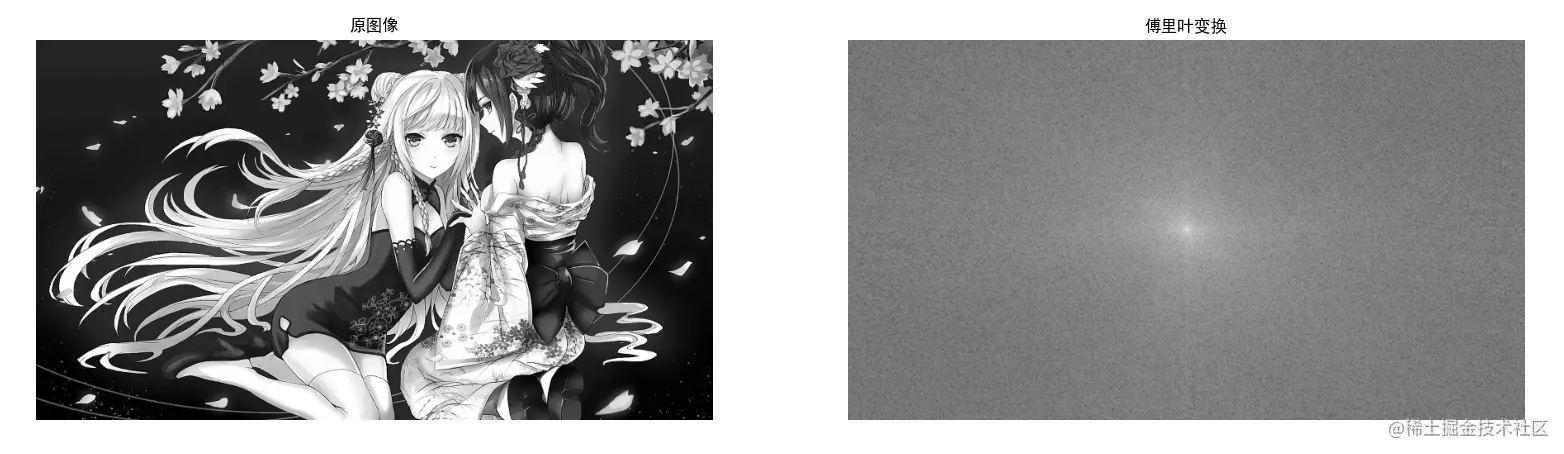Apply the above knowledge , Use OpenCV The function inside uses low-pass filtering for pictures .

``````import cv2 as cv
import numpy as np
import matplotlib.pyplot as plt
plt.rcParams['font.sans-serif'] = ['SimHei']

src = cv.imread("test.jpg", 0)

# OpneCV Fourier transform function
#  The image needs to be processed once float transformation
result = cv.dft(np.float32(src), flags=cv.DFT_COMPLEX_OUTPUT)
#  Move the low frequency of the spectrum from the top left to the center
dft_shift = np.fft.fftshift(result)
#  Spectrum image dual channel complex conversion to  0-255  Section
result = 20 * np.log(cv.magnitude(dft_shift[:, :, 0], dft_shift[:, :, 1]))

rows, cols = src.shape
crow, ccol = rows//2, cols//2

mask = np.zeros((rows, cols, 2), np.uint8)
mask[int(crow-30):int(crow+30), int(ccol-30):int(ccol+30)] = 1
# LPF（ Low pass filtering ）
f_ishift = np.fft.ifftshift(fshift)
img_back = cv.idft(f_ishift)
img_back = cv.magnitude(img_back[:, :, 0], img_back[:, :, 1])

#  Image display
plt.subplot(131), plt.imshow(src, 'gray'), plt.title(' Original image ')
plt.axis('off')
plt.subplot(132), plt.imshow(result, 'gray'), plt.title(' The Fourier transform ')
plt.axis('off')
plt.subplot(133), plt.imshow(img_back, 'gray'), plt.title(' Image after low-pass filtering ')
plt.axis('off')
plt.show()
Copy code ``````High pass filter code and operation effect , Just leave it to yourself .

## Eraser bars

HPF（ High pass filtering ）,LPF（ Low pass filtering ） I hope today's 1 Hours （ It doesn't seem to be enough ） You get something , I'll see you on our next blog ~